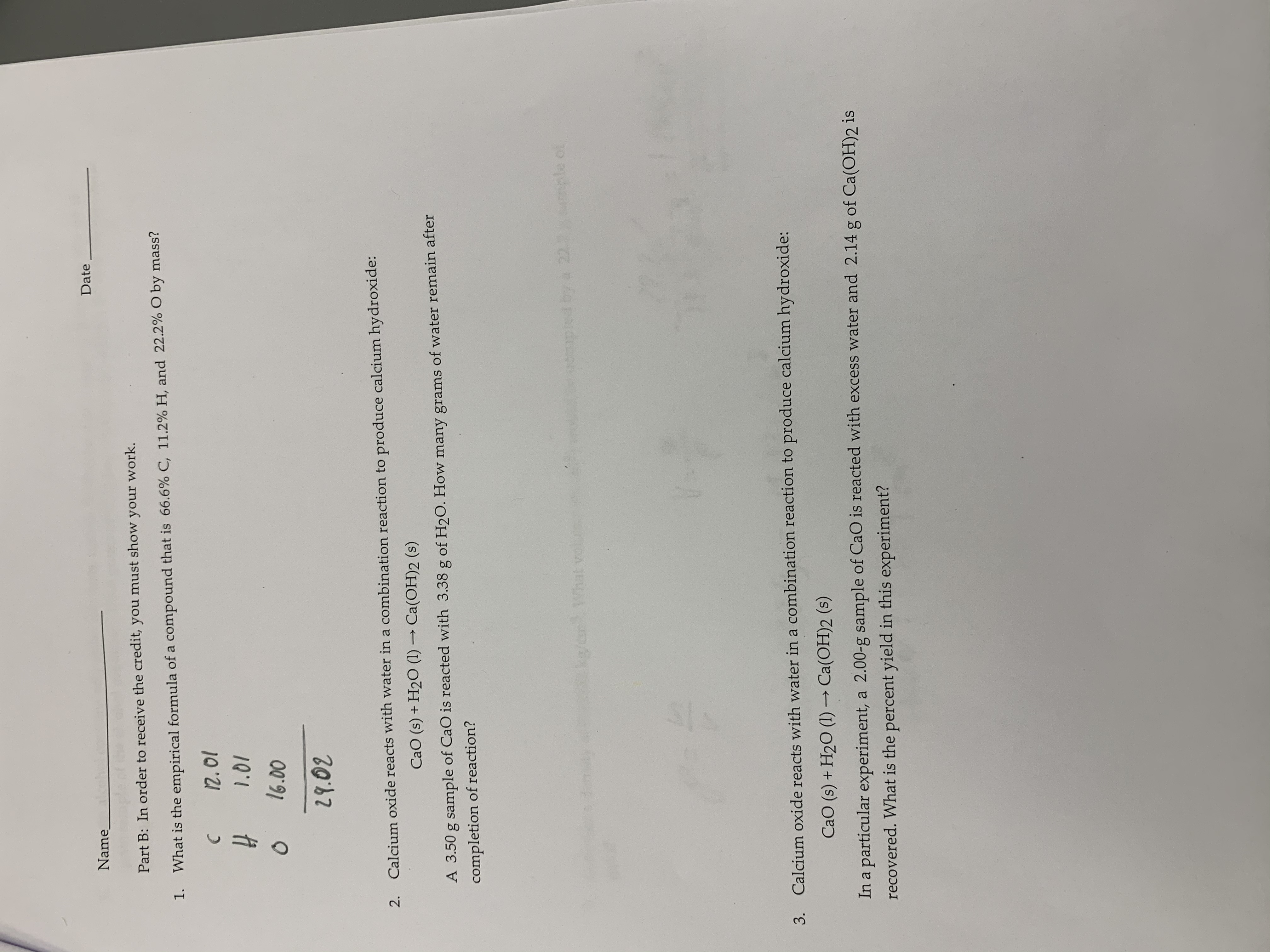# DateNamePart B: In order to receive the credit, you must show your work.What is the empirical formula of a compound that is 66.6% C, 11.2 % H, and 22.2% O by mass?1.12.011.0116.0029.02Calcium oxide reacts with water in a combination reaction to produce calcium hydroxide:2.CaO (s) + H2O (1)Ca(OH)2 (s)A 3.50 g sample of CaO is reacted with 3.38 g of H2O. How many grams of water remain aftercompletion of reaction?apled by a 2211pleCke/arhat voiur4mCalcium oxide reacts with water in a combination reaction to produce calcium hy droxide:3.CaO (s)+ H2O (1)-Ca(OH)2 (s)In a particular experiment, a 2.00-g sample of CaO is reacted with excess water and 2.14 g of Ca(OH)2 isrecovered. What is the percent yield in this experiment?

Question
82 viewshelp_outlineImage TranscriptioncloseDate Name Part B: In order to receive the credit, you must show your work. What is the empirical formula of a compound that is 66.6% C, 11.2 % H, and 22.2% O by mass? 1. 12.01 1.01 16.00 29.02 Calcium oxide reacts with water in a combination reaction to produce calcium hydroxide: 2. CaO (s) + H2O (1)Ca(OH)2 (s) A 3.50 g sample of CaO is reacted with 3.38 g of H2O. How many grams of water remain after completion of reaction? apled by a 2211ple Cke/ar hat voiur 4m Calcium oxide reacts with water in a combination reaction to produce calcium hy droxide: 3. CaO (s)+ H2O (1)-Ca(OH)2 (s) In a particular experiment, a 2.00-g sample of CaO is reacted with excess water and 2.14 g of Ca(OH)2 is recovered. What is the percent yield in this experiment? fullscreen
check_circle

Step 1

The reaction involves,

Step 2

1 mole of CaO needs 2 mole of H2O

0.0624 moles of CaO needs ‘’x’’ mol...

### Want to see the full answer?

See Solution

#### Want to see this answer and more?

Solutions are written by subject experts who are available 24/7. Questions are typically answered within 1 hour.*

See Solution
*Response times may vary by subject and question.
Tagged in

### Chemistry